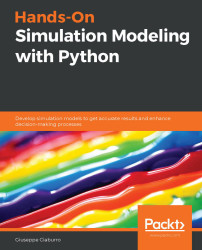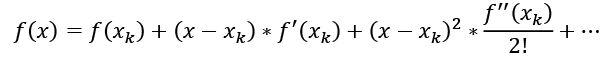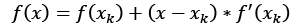•#### Hands-On Simulation Modeling with Python#### Overview of this book

Simulation modeling helps you to create digital prototypes of physical models to analyze how they work and predict their performance in the real world. With this comprehensive guide, you'll understand various computational statistical simulations using Python. Starting with the fundamentals of simulation modeling, you'll understand concepts such as randomness and explore data generating processes, resampling methods, and bootstrapping techniques. You'll then cover key algorithms such as Monte Carlo simulations and Markov decision processes, which are used to develop numerical simulation models, and discover how they can be used to solve real-world problems. As you advance, you'll develop simulation models to help you get accurate results and enhance decision-making processes. Using optimization techniques, you'll learn to modify the performance of a model to improve results and make optimal use of resources. The book will guide you in creating a digital prototype using practical use cases for financial engineering, prototyping project management to improve planning, and simulating physical phenomena using neural networks. By the end of this book, you'll have learned how to construct and deploy simulation models of your own to overcome real-world challenges.
PrefaceSection 1: Getting Started with Numerical SimulationFree Chapter
Chapter 1: Introducing Simulation ModelsChapter 2: Understanding Randomness and Random NumbersChapter 3: Probability and Data Generation ProcessesSection 2: Simulation Modeling Algorithms and TechniquesChapter 4: Exploring Monte Carlo SimulationsChapter 5: Simulation-Based Markov Decision ProcessesChapter 6: Resampling MethodsChapter 7: Using Simulation to Improve and Optimize SystemsSection 3: Real-World ApplicationsChapter 8: Using Simulation Models for Financial EngineeringChapter 9: Simulating Physical Phenomena Using Neural NetworksChapter 10: Modeling and Simulation for Project ManagementChapter 11: What's Next?Other Books You May Enjoy# Facing the Newton-Raphson method

Newton's method is the main numerical method for the approximation of roots of nonlinear equations. The function is linearly approximated at each iteration to obtain a better estimate of the zero point.

## Using the Newton-Raphson algorithm for root-finding

Given a nonlinear function f and an initial approximation x0, Newton's method generates a sequence of approximations {xk} k > 0 by constructing, for each k, a linear model of the function f in a neighborhood of xk and approximating the function with the model itself. This model can be constructed starting from Taylor's development of the function f at a point x belonging to a neighborhood of the iterated current point xk, as follows:Truncating Taylor's first-order development gives us the following linear model:The previous equation remains valid in a sufficiently small neighborhood of xk.

Given x0 as the initial data, the first iteration consists of...